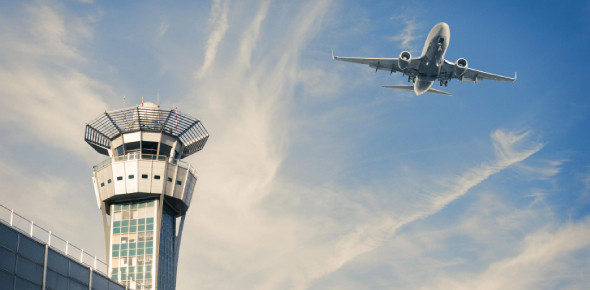# Take This Air Navigation Quiz - 1

19 Questions | Attempts: 1138
ShareSettingsNot just anyone can get to navigate an airplane safely without any studying. If you are studying pilot you need to ensure that you keep revising and refreshing you memory on things already studies. Take up the quiz below and test yourself, look out for new ones that will help you ace your exams.

• 1.
In a triangle ABC, a=421.9, c=321.6, ∟A = 65deg12', find angle C.
• A.

52deg23'

• B.

62deg12'

• C.

43deg47'

• D.

23deg10'

• 2.
The spacing of the parallels along the meridian is given by the following formulae
• A.

Difference of latitude ---------------------------- X 2πr 360

• B.

360 X 2πr X difference of latitude

• C.

Difference of longitude ----------------------------- X 2πr 360

• D.

360 X 2πr X difference of longitude

• 3.
ICAO's definition of a nautical mile is that it is a measure of distance of _____
• A.

1258 kms

• B.

1852 meters

• C.

8521 meters

• D.

1825 kms

• 4.
What is the distance from Kolkatta (30degN 90degE) to New Orleans, USA (30degN 90degW) over the north pole?
• A.

7200nm

• B.

2300nm

• C.

6500nm

• D.

None of the above

• 5.
Give the ch long (change of longitude) between160degW and 158degE
• A.

2degW

• B.

2degE

• C.

42degW

• D.

56degW

• 6.
GC bearing of Q from P is 060deg, CA = 4deg; if the two places are in the northern hemisphere, what is the a) RL bearing of Q from P?b) RL bearing of P from Q?c) GC bearing of P from Q?
• A.

A) 089deg b) 270deg c) 247 deg

• B.

A) 064deg b) 244deg c) 258deg

• C.

A) 244deg b) 247deg c) 064deg

• D.

A) 063deg b) 214deg c) 248deg

• 7.
An aircraft takes off from 47deg30'S 178deg30'W and flies a rhumb line track of 270deg for 224 nm. What is its new position? (Sec 47deg30' = 1.48)
• A.

47deg30'E 170deg59'S

• B.

147deg30'S 17deg40'E

• C.

47deg30'S 175deg23'E

• D.

47deg30'S 175deg59'E

• 8.
A flight is planned from 40degS 110degE to 40degS 102degE. Give the distance in kilometers if the flight is taking place along the rhumb line track. (Cos 40deg = 0.766)
• A.

598.5 km

• B.

680 km

• C.

681.5 km

• D.

686 km

• 9.
An aircraft takes 15 minute 12 seconds to cover the distance 6.6cm between A and B on a chart having a scale of 1:2 000 000. Calculate the aircraft's ground speed in knots.
• A.

254.2

• B.

132

• C.

281.3

• D.

244.7

• 10.
The distance flown by an aircraft in 40seconds at a ground speed of 480kt is shown on a chart by a straight line, 1.4 inches long. Give the scale of the chart in centimeters to kilometers.
• A.

1 cm to 38.9km

• B.

1 cm to 2.778km

• C.

1 cm to 3.89 km

• D.

1 cm to 23km

• 11.
On a Mercator, two meridians one degree apart are spaced at a distance of 7.62 cm. What is the scale on this chart at 48degN (Sec 48deg = 1.49, cos 48deg = 0.67)
• A.

1 : 977664

• B.

1 : 652314

• C.

1 : 97466

• D.

1 : 235689

• 12.
At 60degN, a 30 cm on the chart measures a ground distance of 390nm. What is the scale of the chart at the equator?
• A.

1: 2451368

• B.

1: 2350000

• C.

1: 4810000

• D.

1: 2333125

• 13.
A Mercator has a scale of 1:1000 000 at the eqator. On this scale, two positions A and B, both on 54degN are shown 10 inches apart. What is the difference in longitude between them?
• A.

2deg16.2'

• B.

2deg17.1'

• C.

1deg16.2'

• D.

1deg17.1'

• 14.
Aircraft on heading 270deg(T) in DR position 46degN 84degW obtains relative bearing 239deg from station 40degN 81degW. What would you plot?
• A.

331deg

• B.

231deg

• C.

352deg

• D.

335deg

• 15.
Aircraft in DR position 69deg30'S 65degW obtains QDM 277deg from station at 71degS 70degW variation at a/c position 19degW; mean variation 22degW, variation at station 25degW. What would you plot?
• A.

078deg

• B.

069deg

• C.

075deg

• D.

072deg

• 16.
The transverse Mercator  Projection is
• A.

Conformal and convergency is correct everywhere

• B.

Conformal and convergency is correct along all lines(great circles) at 90 deg to the central and anti-meridians

• C.

Not conformal and convergency is not correct anywhere

• 17.
On a oblique Mercator,scale error is negligible for practical aviation purposes(ie less than 2 %)within a distance from the datum great circle of
• A.

70 nm

• B.

700 nm

• C.

1700 nm

• 18.
Hdg(T) is 258 deg,Convergence 20 deg E;what is Hdg(G)?
• A.

278

• B.

280

• C.

258

• D.

269

• 19.
An aircraft has a grid heading of 310 deg using a chart based on grid datum of 40 deg W. If the variation is 10 deg E, n=0.8 and Hdg(M) 340 deg, what is the aircraft's longitude
• A.

10 deg S

• B.

9 degW

• C.

10degE

• D.

9 degE

## Related TopicsBack to top
×

Wait!
Here's an interesting quiz for you.